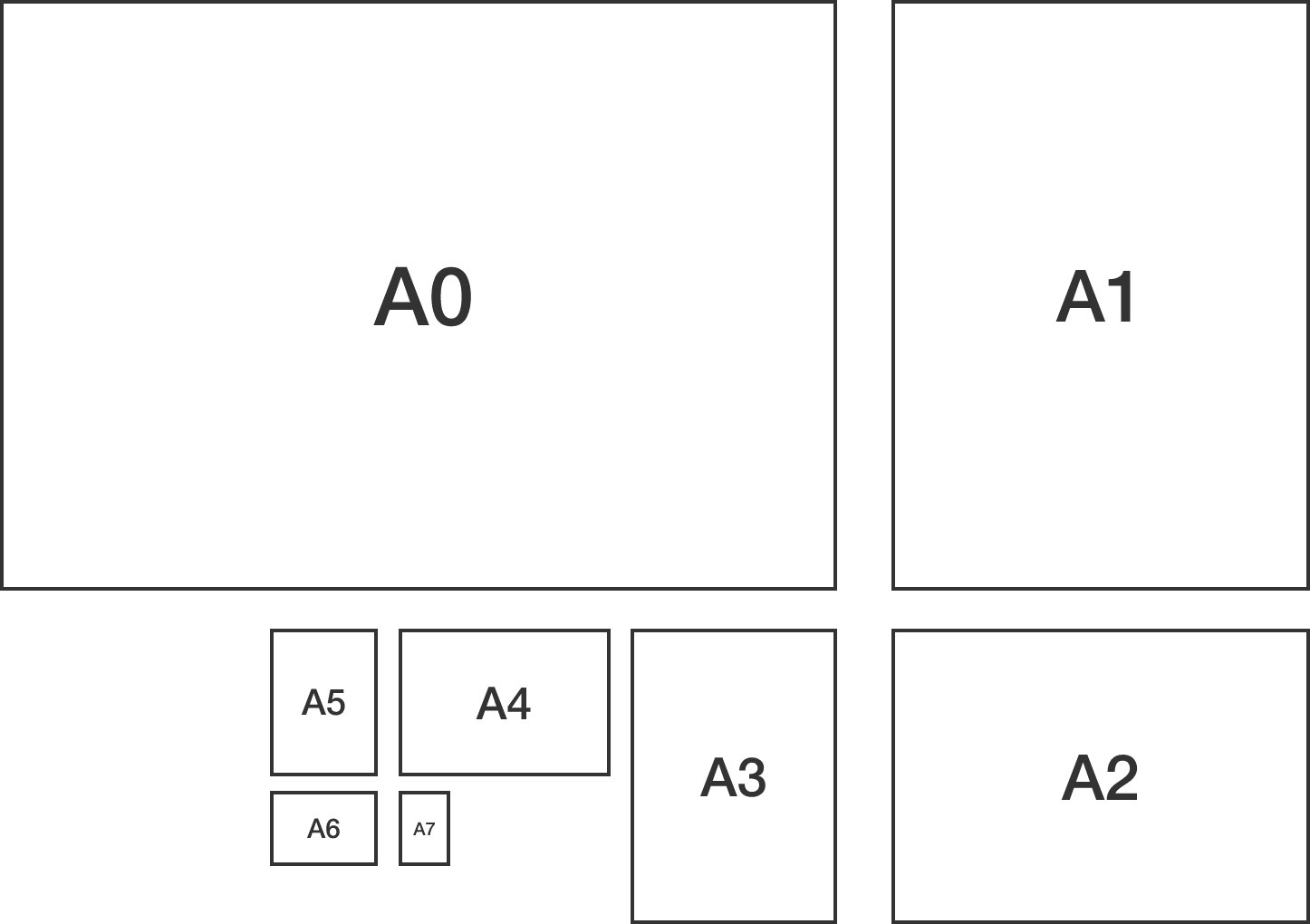# Standard paper

Geometry Level 3

The A-series of paper sizes used in Europe have the following properties:

• A sheet of A0 paper is a rectangle with an area of precisely one square meter. Its length (the long side) is less than twice its width.

• When A0 paper is cut in half across its long side, two sheets of A1 paper are formed. Likewise, when A1 paper is cut in half across its long side, A2 paper is formed, and so on.

• All rectangles in the series (A0, A1, A2, ...) are similar.What is the length of a sheet of A6 paper, in meters?

×